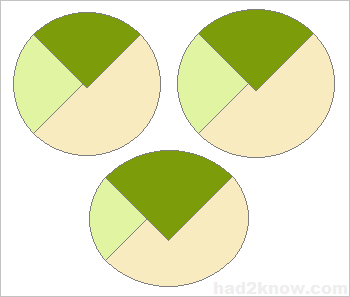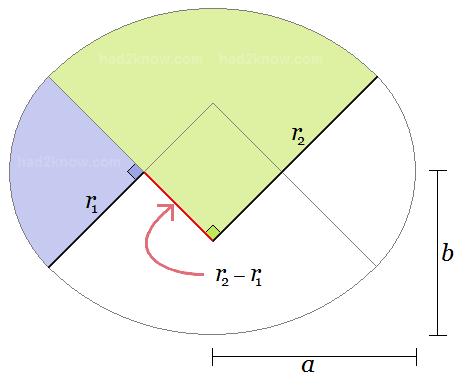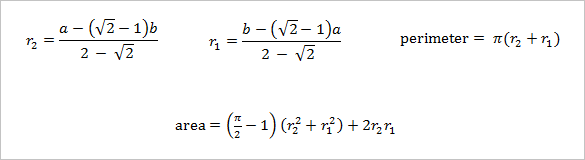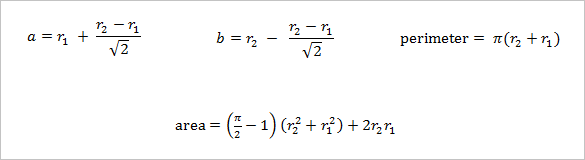# Quarter Circle Arc Ellipse Approximation

Ellipse Approximation Calculator
major/minor axes known, arc radii unknown
half-major axis =
half-minor axis =
arc radii known, major/minor axes unknown
Area =
Perimeter =

In manufacturing and design it is often easier to create ellipse approximations rather than true ellipses. Several methods of creating pseudo-ellipses use circular arcs.

For example, two pairs of quarter circle arcs can be used to make a figure that looks very much like a true ellipse. The size difference between the arcs determines the shape's eccentricity.Denote the length of the half-major axis by a, the half-minor axis by b, the longer arc radius by r2, and the shorter arc radius by r1. If you know both a and b, then you can determine r2 and r1.

Likewise, if you know r2 and r1, you can solve for a and b. All of the necessary formulas are given below, or you can use the convenient calculator above.

### Calculating r2 and r1 from a and b

Given dimensions a and b, where ab and a ≤ (1+√2)b, you can find the values of r2 and r1 as well as the perimeter and area with the equations### Calculating a and b from r2 and r1

Given r2 and r1, you can find the values of a and b as well as the perimeter and area with the equations